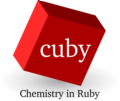back to Interfaces

# QMMM interface

Difference between revisions from 2010/12/02 21:35 and 2010/12/02 11:54.
```Two-level hybrid calculation (QM/MM, QM/QM', ...) using a subtractive scheme similar to ONIOM^[1,2^].
Two-level hybrid calculation (QM/MM, QM/QM', ...) using a subtractive scheme similar to ONIOM^[1,2^]. The [QMMM tutorial] shows how to setup a calculation.

!Keywords
* qmmm_core
* qmmm_qm_charge
* qmmm_cut_bonds
* qmmm_rename_residues
* qmmm_geometry_only
* polarized
* qmmm_remove_charges
* microiterations

The methods of calculations are specified in subsections
* '''qmmm_method_hi'''
* '''qmmm_method_lo'''
The subsections
* '''qmmm_method_lo_mmpart'''
* '''qmmm_method_lo_qmpart'''
allows to modify the MM calculations of the complete system and QM region separately. Keywords used here are added to or override the ones in qmmm_method_lo.

!References
^[1^] M. Svensson, S. Humbel, R. D. J. Froese, T. Matsubara, S. Sieber, K. Morokuma, ONIOM: A multilayered integrated MO+MM method for geometry optimizations and single point energy predictions. A test for Diels-Alder reactions and Pt(P(t-Bu)(3))(2)+H-2 oxidative addition, Journal of Physical Chemistry 100(50), 19357-19363, '''1996'''
^[2^] S. Dapprich, I. Komaromi, K. S. Byun, K. Morokuma, M. J. Frisch, A new ONIOM implementation in Gaussian98. Part I. The calculation of energies, gradients, vibrational frequencies and electric field derivatives, J. Mol. Struct. Theochem 461, 1-21, '''1999'''

```